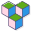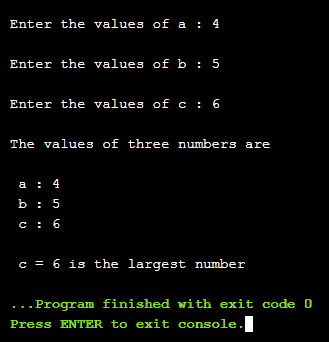CppBuzz# C program to find largest among three numbers

This C program takes input from user and finds out the largest number.

``````#include <stdio.h>
#include <conio.h>

int main()
{
//declare three variables
int a, b, c;

printf("\n\nEnter the values of a : ");
scanf("%d",&a);
printf("\nEnter the values of b : ");
scanf("%d",&b);
printf("\nEnter the values of c : ");
scanf("%d",&c);

printf("\nThe values of three numbers are \n");
printf("\n a : %d",a);
printf("\n b : %d",b);
printf("\n c : %d\n",c);

if(a>b)
{
if(a>c)
printf("\n a = %d is the largest number",a);
else
printf("\n c = %d is the largest number",c);
}
else
{
if(c>b)
printf("\n c = %d is the largest number",c);
else
printf("\n b = %d is the largest number",b);
}

//hold the screen
getchar();

return 0;
}
```
```

## The output is 6 if user enters 4, 5, 6